# Calculating Speed Velocity Acceleration and Kinetic Energy FORMULAS

• Slides: 18
Download presentation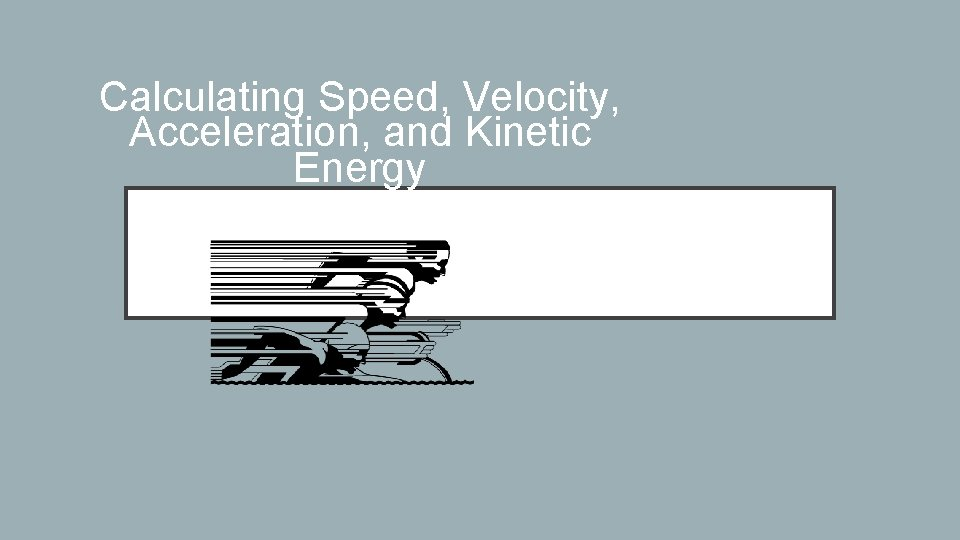Calculating Speed, Velocity, Acceleration, and Kinetic EnergyFORMULAS TO REMEMBER • Speed = Distance / time • Average Speed = Total distance / total time • Velocity = Distance / time + direction • Acceleration= Final Velocity – Initial Velocity • time • Kinetic Energy= ½ mass x speed 2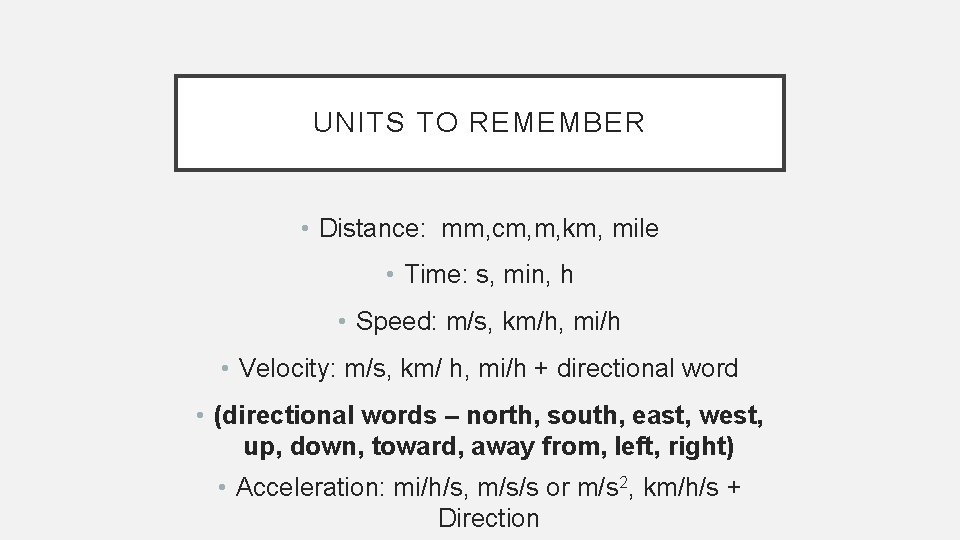UNITS TO REMEMBER • Distance: mm, cm, m, km, mile • Time: s, min, h • Speed: m/s, km/h, mi/h • Velocity: m/s, km/ h, mi/h + directional word • (directional words – north, south, east, west, up, down, toward, away from, left, right) • Acceleration: mi/h/s, m/s/s or m/s 2, km/h/s + Direction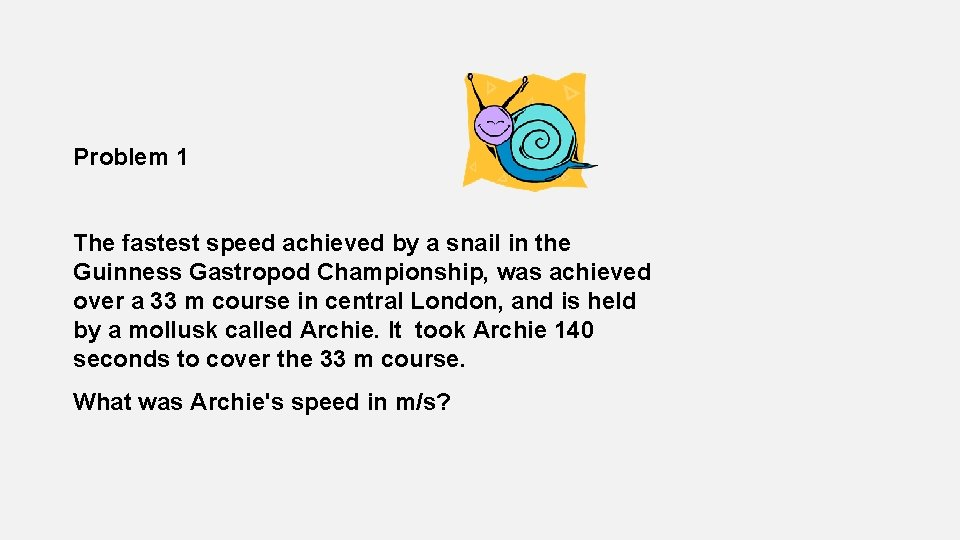Problem 1 The fastest speed achieved by a snail in the Guinness Gastropod Championship, was achieved over a 33 m course in central London, and is held by a mollusk called Archie. It took Archie 140 seconds to cover the 33 m course. What was Archie's speed in m/s?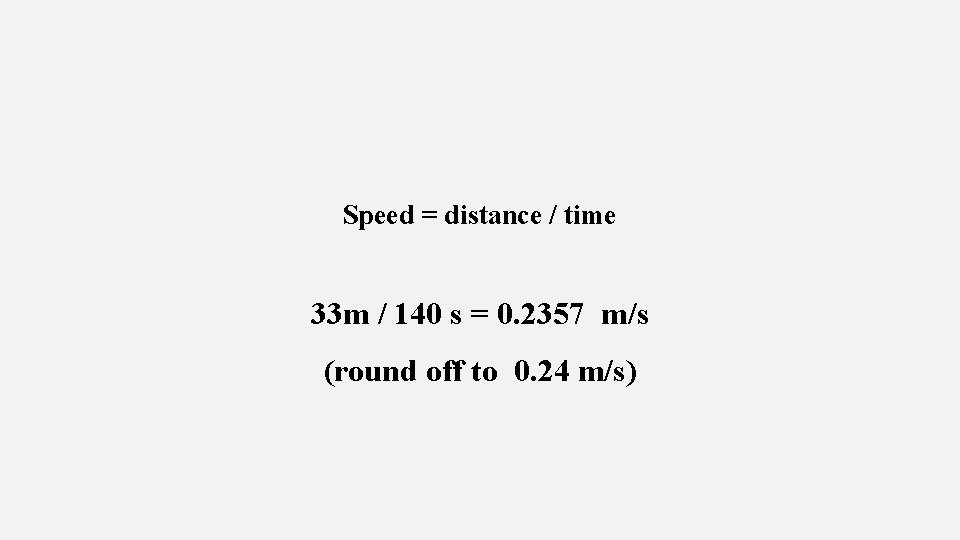Speed = distance / time 33 m / 140 s = 0. 2357 m/s (round off to 0. 24 m/s)A driver, not anticipating an accident, can apply the brakes fully in about 0. 5 seconds. How far would a car driving down the freeway at 30 m/s travel in this time? S=d/t d=tx. S t=d/S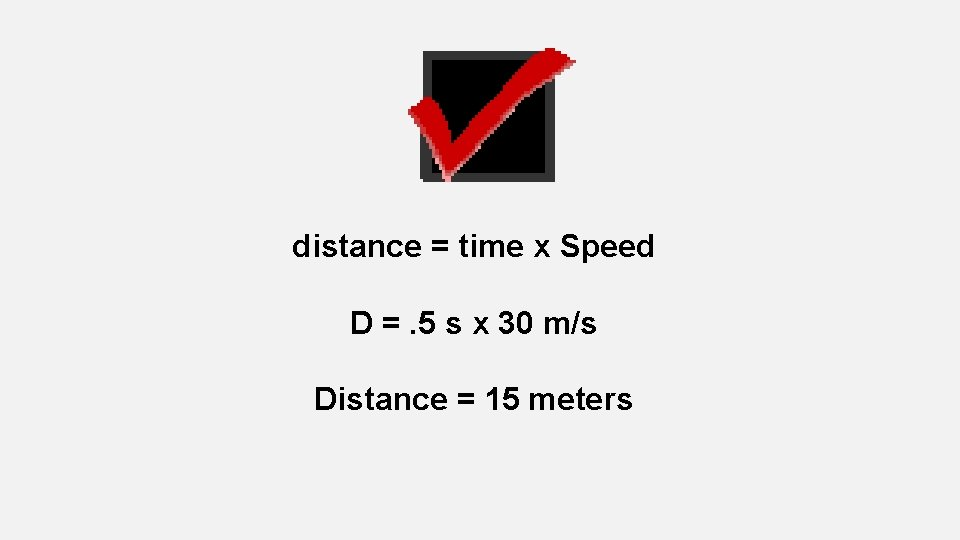distance = time x Speed D =. 5 s x 30 m/s Distance = 15 meters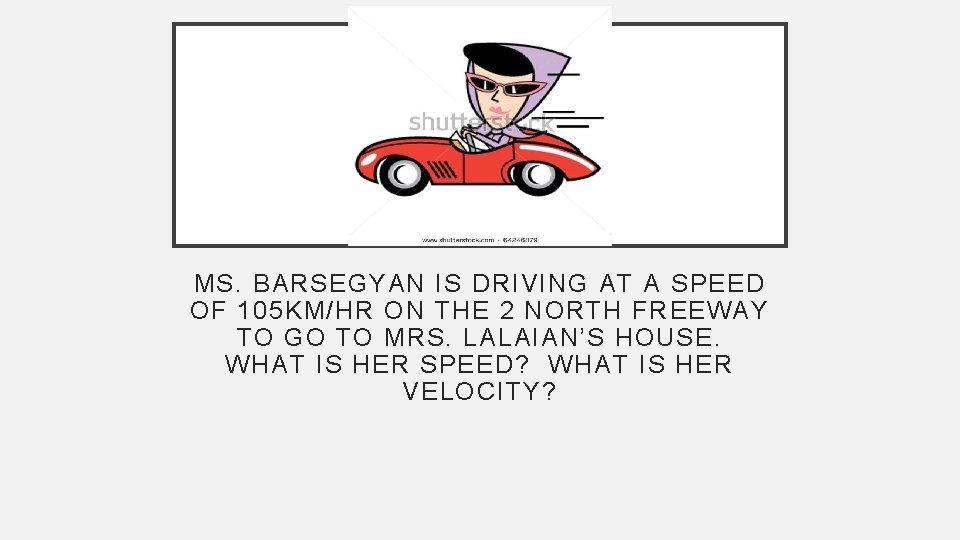MS. BARSEGYAN IS DRIVING AT A SPEED OF 105 KM/HR ON THE 2 NORTH FREEWAY TO GO TO MRS. LALAIAN’S HOUSE. WHAT IS HER SPEED? WHAT IS HER VELOCITY?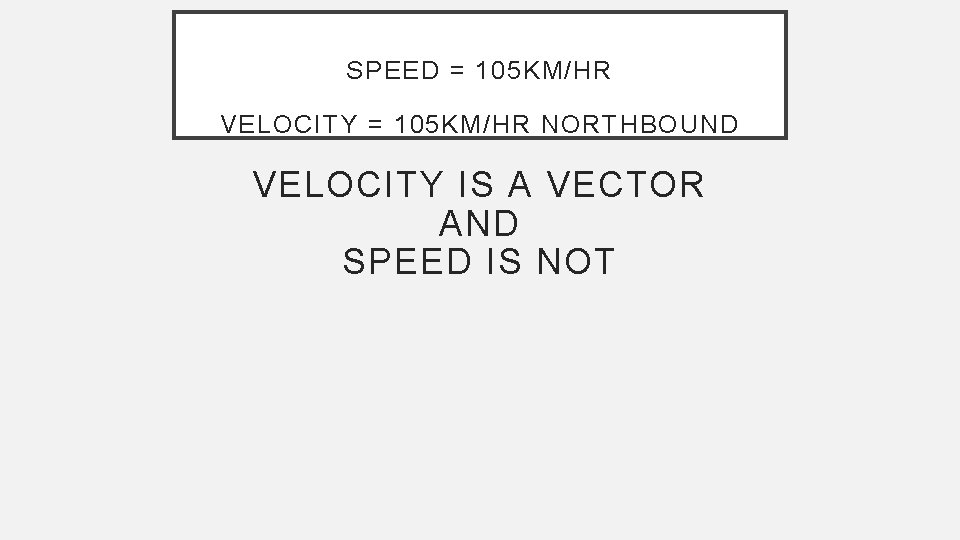SPEED = 105 KM/HR VELOCITY = 105 KM/HR NORTHBOUND VELOCITY IS A VECTOR AND SPEED IS NOTMRS. CERVANTES RUNS 30 METERS IN 5 SECONDS TO 1 ST BASE. SHE WAITS FOR 60 SECONDS FOR THE NEXT BATTER, WHO HITS A HOMERUN. MRS. CERVANTES RUNS THE REMAINING 70 M TO THE 2 ND BASE, 3 RD BASE AND HOME IN 10 SECONDS. WHAT IS HER AVERAGE SPEED BACK TO THE BATTER BOX?30 M+70 M 5 S+60 S+10 S= 100 M = 75 S 1. 33 M/S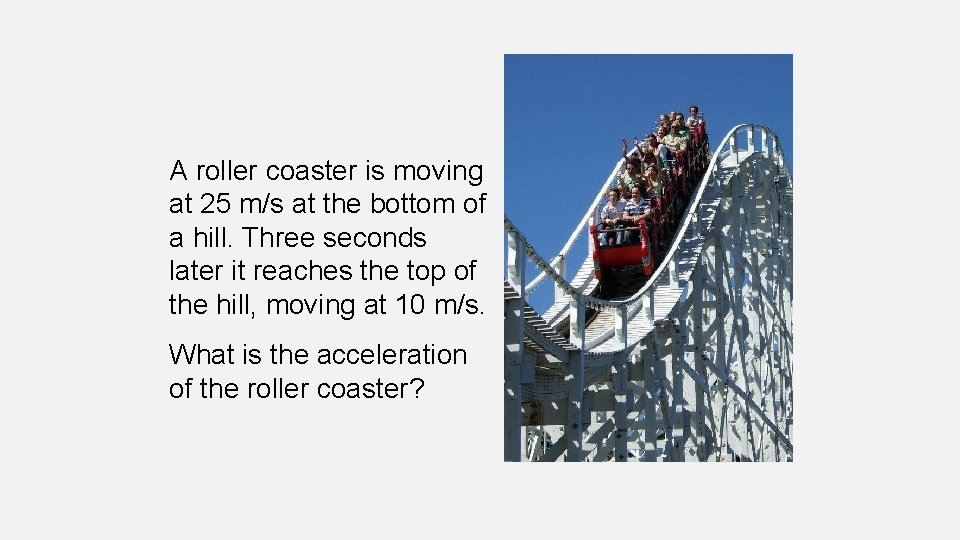A roller coaster is moving at 25 m/s at the bottom of a hill. Three seconds later it reaches the top of the hill, moving at 10 m/s. What is the acceleration of the roller coaster?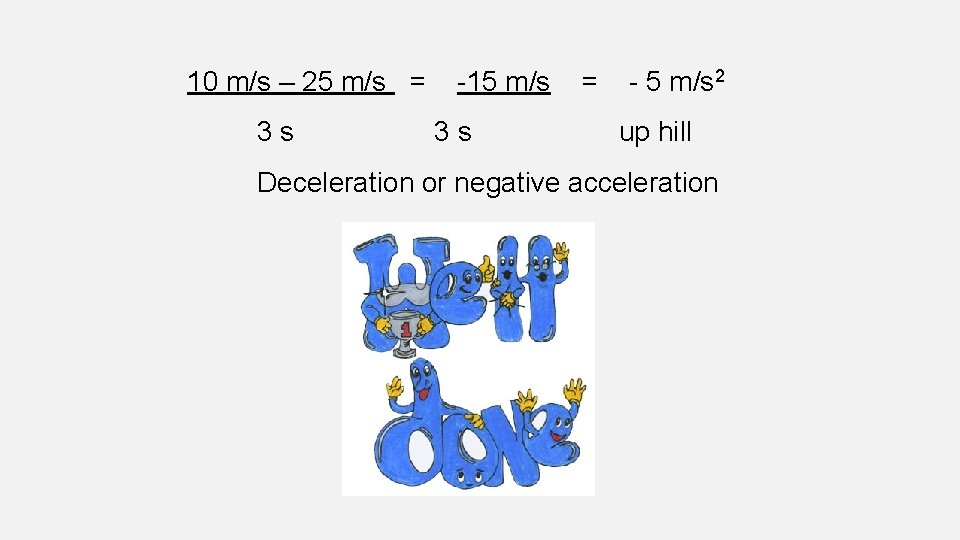10 m/s – 25 m/s = -15 m/s = - 5 m/s 2 3 s up hill Deceleration or negative accelerationA driver starts his parked car and within 5 seconds reaches a velocity of 55 km/h as he travels east. What is his acceleration?Good Job, Man! 55 km/h – 0 km/h = 55 km/h = 11 km/h/s 5 sec 5 s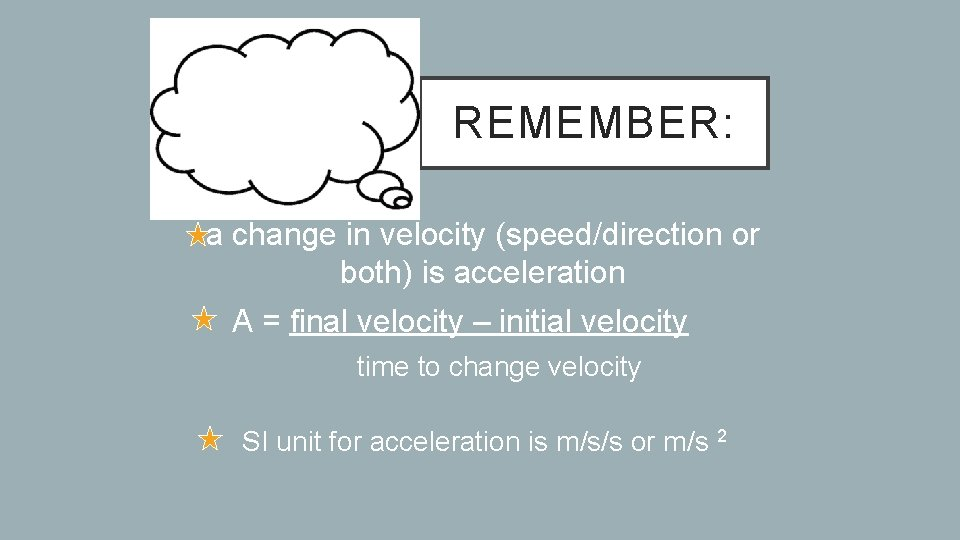REMEMBER: a change in velocity (speed/direction or both) is acceleration A = final velocity – initial velocity time to change velocity SI unit for acceleration is m/s/s or m/s 2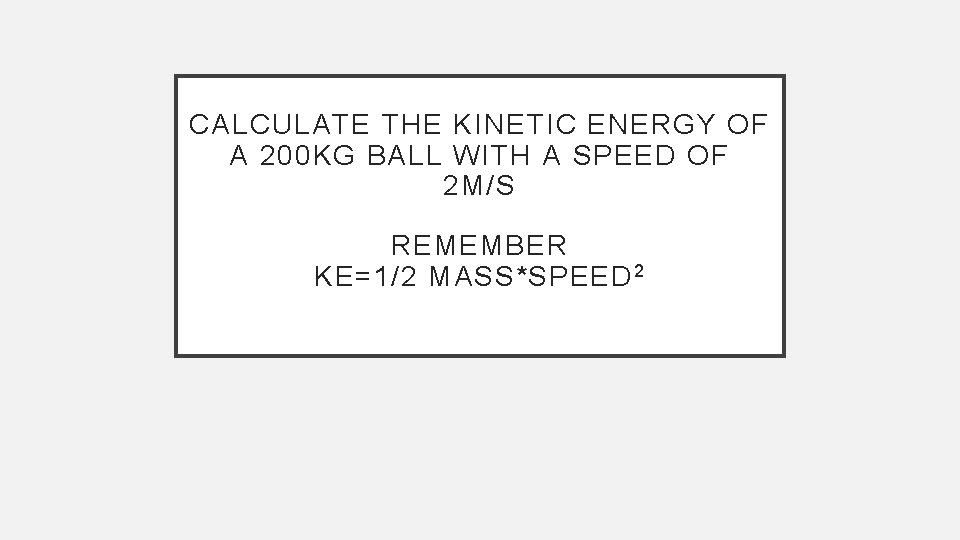CALCULATE THE KINETIC ENERGY OF A 200 KG BALL WITH A SPEED OF 2 M/S REMEMBER KE=1/2 MASS*SPEED 2KE= ½*200 KG*(2 M/S) 2 =400 J